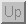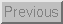Next: 4.6 Capacitance to Voltage Up: 4 Signal Conditioning Previous: 4.4 Current to Voltage

## 4.5 Resistance to Voltage

### 4.5.1 Motivation

Many sensors exhibit a change electrical resistance in response to the quantity that they are trying to measure. Some examples include force sensing resistors which decrease their resistance when a force is applied, thermistors which change resistance as a function of the temperature and carbon microphones which alter their resistance in response to changing acoustical pressure. In all these cases, one must be able to convert the resistance of the device into a usable voltage which can be read by the analog to digital converters. Following are some circuits which perform these measurements along with some examples of sensors that were described in previous sections.

### 4.5.2 Circuits

There are two ways to convert resistance of a sensor to a voltage. The first, and simplest way is to apply a voltage to a resistor divider network composed of a reference resistor and the sensor as shown in Figure 32.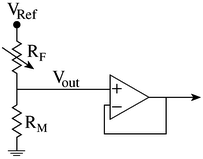Figure 32: Resistance to Voltage

The voltage that appears across the sensor (or the reference resistor) is then buffered before being sent to the ADC. The output voltage is given by: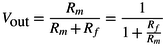The problem with this method of measuring resistance is that the amplifier is amplifying the entire voltage measured across the sensor. It would be much better to amplify only the change in the voltage due to a change in the resistance of the sensor. This can be accomplished using a bridge as shown in Figure 33.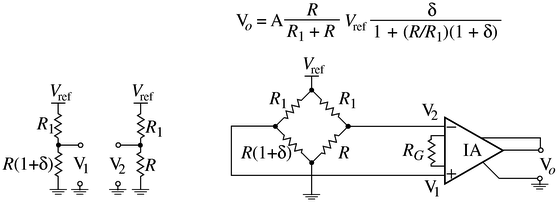Figure 33: A Resistance Bridge Connected to an Instrumentation Amplifier (IA)

If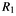is set equal to R, then the approximate output of this circuit is: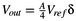Where A is the gain of the IA and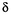is the change in the resistance of the sensor corresponding to some physical action. Notice in this equation that the gain can be set quite high because only the change in voltage caused by a change in the sensor resistance is being amplified.

### 4.5.3 Example

FSRFigure 34: An FSR in a voltage divider configuration

The most basic method of interfacing to an FSR is depicted in Figure 34. In this configuration an FSR is used in a voltage divider configuration as described previously. In this case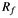from Figure 32 is the force sensing resistor. An increase in force results in a decrease in the value of, and hence an increase in the output voltage. This configuration will not produce a voltage which is a linear function of force. If a linear characteristic is desired, then one must use a different configuration, or compensate for the actual response in software once the voltage data is acquired.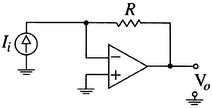Figure 35: Measuring the current through an FSR

An alternative to the above implementation is to measure the current through the device, and then use an op-amp circuit to convert the current to a voltage. The current through the FSR is proportional to the conductance which is in turn proportional to the force, hence current is proportional to force. Moreover, within the appropriate region, this is a linear relationship. Figure 35 depicts an op-amp in a current to voltage configuration. What is shown here is simply the output of the FSR connected to the inverting input of the inverting amplifier described previously. The output voltage,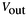is given by: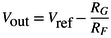Since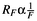,is linearly proportional to force. Since the non-inverting terminal of the op-amp is grounded, the inverting terminal is effectively at ground, hence the current through the FSR is given by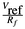, hence the above expression can be written as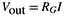.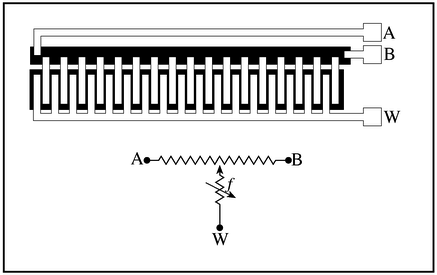Figure 36: Linear potentiometer configuration of an FSR capable of measuring both force and position.

Standard FSRs are two terminal devices which measure force. Another configuration offered by Interlink is a three terminal linear potentiometer. Depending upon the circuitry it is hooked up to, this device can be used to measure either force or position. The physical layout of the device and a schematic representation of the device are given in Figure 36.

In order to understand how it measures the position of contact, first imagine that the force sensing resistive element is simply a wire. When pressure is exerted somewhere along the length of the device, contact is made. By measuring the amount of the resistance from either of the ends to one of the terminals one can determine the location at which the potentiometer has been pressed. One way to do this is to apply a voltage across the potentiometer. When the device is pressed, a connection is established which effectively creates a voltage divider. The voltage which appears at this terminal will be proportional to the position of contact. In our case, we do not have a wire, but rather a resistor, hence any current flowing through the resistor will cause a voltage drop. Furthermore, we cannot know the amount of the voltage drop since the resistance is unknown. One way around this dilemma is to sense the voltage while drawing as little current as possible. In order to do this, a buffer circuit, like the one described previously, is employed. This is summarized in Figure 37.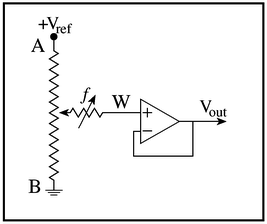Figure 37: High input impedance measuring system used to measure position.

One way to measure the applied force is to apply a voltage to only one end of the potentiometer while leaving the other end open. Using the voltage divider circuit discussed earlier, one can measure the series combination of the force sensing resistive element and an unknown amount of resistance due to the section of the linear potentiometer. This circuit is shown in Figure 38. The amount of uncertainty can be reduced by tying both ends to the voltage supply. A more sophisticated approach is to implement a circuit that can switch between the two measurements schemes just discussed. Furthermore, if this is done fast enough, one can obtain both measurements almost simultaneously, and use the position measurement to compensate for the unknown resistance in the above scheme.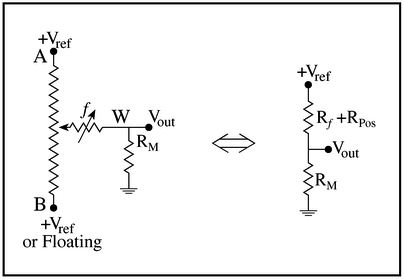Figure 38: Circuit used to measure force with a linear FSR potentiometer.Next: 4.6 Capacitance to Voltage Up: 4 Signal Conditioning Previous: 4.4 Current to Voltage

Tim Stilson
Thu Oct 17 16:32:33 PDT 1996